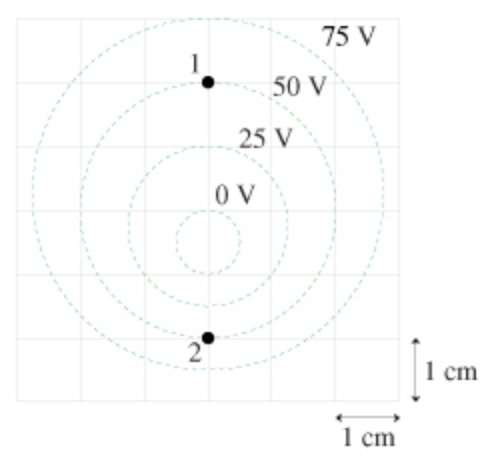In: Physics

# Determine the magnitude and direction of the electric field at point 1 in the figure(Figure 1).

Figure 1Part A

Determine the magnitude and direction of the electric field at point 1 in the figure(Figure 1).

 E1→=(2500V/m,up) E1→=(7500V/m,up) E1→=(3750V/m,down) E1→=(2500V/m,down)

Part B

Determine the magnitude and direction of the electric field at point 2 in the figure.

 E2→=(2500V/m,up) E2→=(3750V/m,down) E2→=(7500V/m,down) E2→=(5000V/m,up)

## Solutions

##### Expert Solution

Part A

The relation between electric field and potential is, $$E=-\frac{\Delta V}{d}$$

The magnitude of the electric field at point 1 is, $$E_{1}=\left|-\left(\frac{50 \mathrm{~V}-0 \mathrm{~V}}{2 \mathrm{~cm}}\right)\right|$$

$$=\left(\frac{50 \mathrm{~V}-0 \mathrm{~V}}{2 \mathrm{~cm}}\right)$$

$$=\frac{50 \mathrm{~V}}{2 \times 10^{-2} \mathrm{~m}}$$

$$=2500 \mathrm{~V} / \mathrm{m}$$

The direction of the electric field is from high potential to low potential, so the direction of the electric field at point 1 is downwards.

Part B The magnitude of the electric field at point 2 is, $$E_{1}=\left|-\left(\frac{50 \mathrm{~V}-0 \mathrm{~V}}{1 \mathrm{~cm}}\right)\right|$$

$$=\left(\frac{50 \mathrm{~V}-0 \mathrm{~V}}{1 \mathrm{~cm}}\right)$$

$$=\frac{50 \mathrm{~V}}{1 \times 10^{-2} \mathrm{~m}}$$

$$=5000 \mathrm{~V} / \mathrm{m}$$

The direction of the electric field is from high potential to low potential, so the direction of the electric field at point 1 is upwards.

Part B

The magnitude of the electric field at point 2 is,

\begin{aligned} E_{1} &=\left|-\left(\frac{50 \mathrm{~V}-0 \mathrm{~V}}{1 \mathrm{~cm}}\right)\right| \\ &=\left(\frac{50 \mathrm{~V}-0 \mathrm{~V}}{1 \mathrm{~cm}}\right) \\ &=\frac{50 \mathrm{~V}}{1 \times 10^{-2} \mathrm{~m}} \\ &=5000 \mathrm{~V} / \mathrm{m} \end{aligned}

The direction of the electric field is from high potential to low potential, so the direction of the electric field at point 1 is upwards.